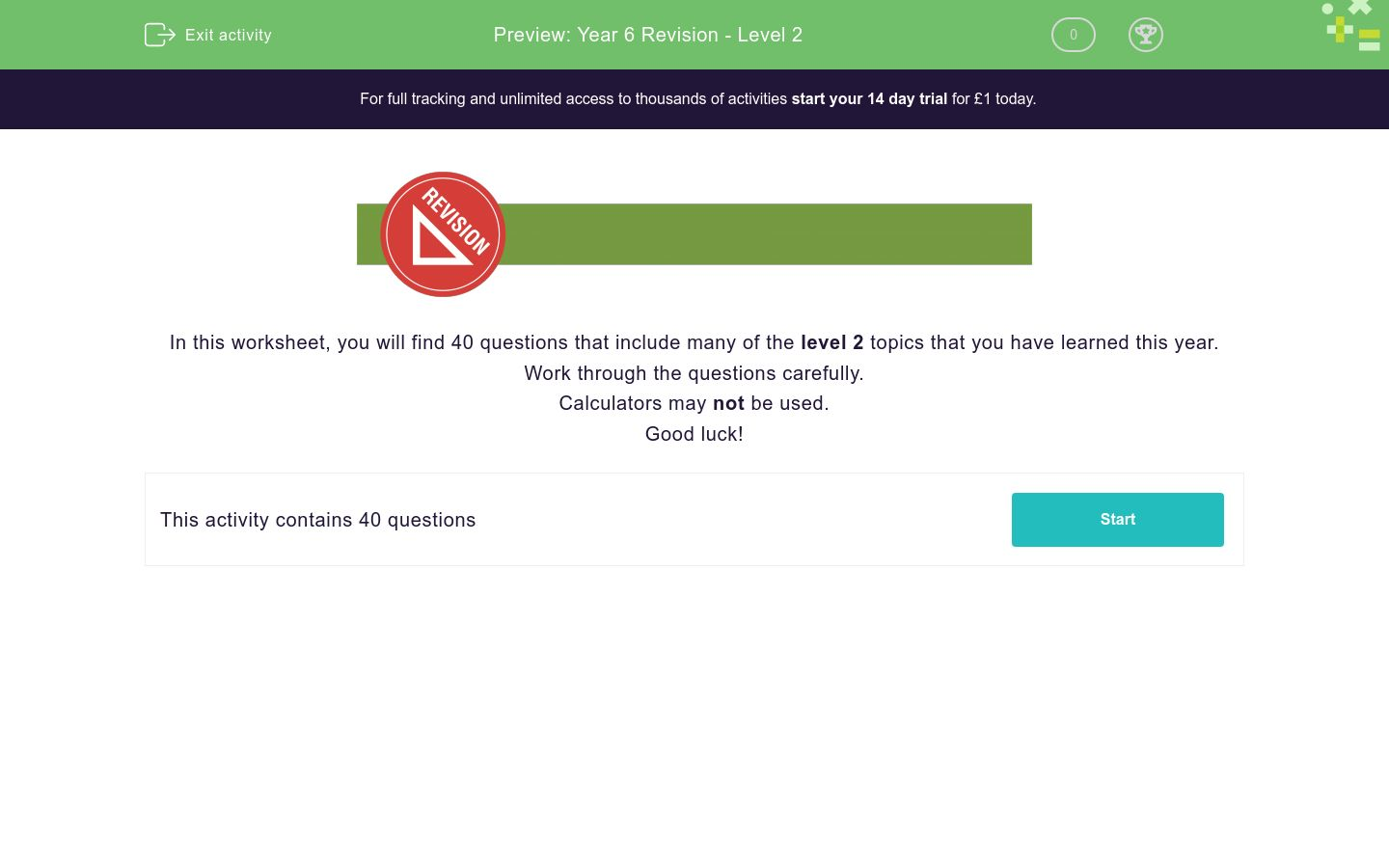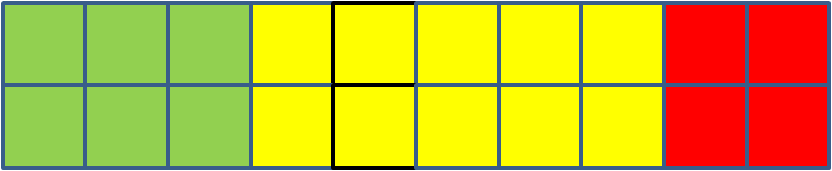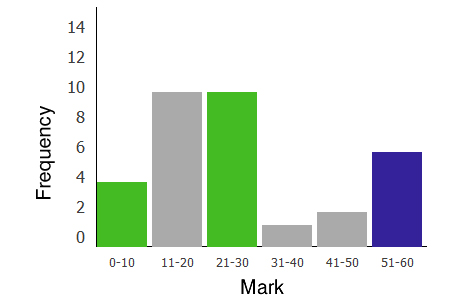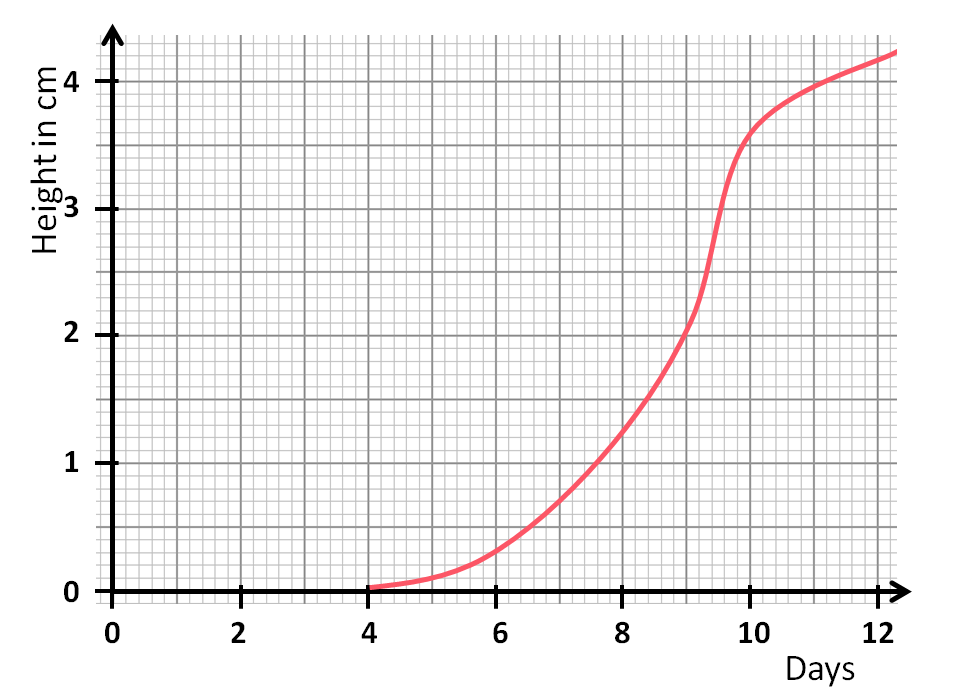# Year 6 Revision - Level 2

This is a level 2 revision exercise to help prepare for Year 6/end of Key Stage 2 tests.Key stage:  KS 2

Curriculum topic:   Revision

Curriculum subtopic:   Year 6 Revision

Difficulty level:### QUESTION 1 of 10In this worksheet, you will find 40 questions that include many of the level 2 topics that you have learned this year.

Work through the questions carefully.

Calculators may not be used.

Good luck!

Write this number using figures:

Three hundred and fourteen thousand and sixty-two

How many ten thousands are there in the number:

4 521 637

1

2

3

4

5

6

7

Select all the numbers which are bigger than 400 000.

50 000

550 001

350 909

400 040

5 420 000

Round to the nearest hundred thousand:

3 456 011

Select all the prime numbers in this list.

13

15

35

37

27

29

23

64 - 26 + 12

Subtract:

3653 - 1974

(Use a piece of paper, and set the numbers out in a column subtraction.)

Use long multiplication to work out:

1616 × 34

Write down two numbers between 20 and 50 that are multiples of both 3 and 7.

 1st number 2nd number

Use long division to work out:

6594 ÷ 21

Write down the number which is halfway between:

540 100 and 640 300

Work out:

750 → + 9× 2- 8÷ 2 → ?

Work out:

(25 + 3) ÷ (8 - 1)

Choose all the fractions which are equivalent to the decimal:

0.375

375/100

375/1000

75/200

75/250

15/40

3/8

5/8

Convert the following percentage to a decimal:

90%

(Do not type any more digits than are needed.)

Work out half of:

0.69

Work out:

2.603 + 5.7 - 4

Work out:

7.89 × 6

Work out:

2.6 ÷ 4

Look at the shading in this rectangle.30%

6%

60%

Work out:

20% of 250

Work out the following, remembering to give your answer in its lowest terms, e.g. 7/10.

 3 - 7 5 20

Work out the following, reducing your answer to its simplest form if you can.

 8 ÷ 6 9

3/5 of a DVD is used to record a film.

What percentage of the DVD remains unrecorded?

(Just write the number.)

These two shapes are similar.

State the missing length in cm.

(Just write the number.)If 24 beads are used in the bracelet, how many blue beads are used?

I think of a number.

I divide it by 5 and add 2.

Then I add this to twice the original number.

I get 24.

Write this using algebra.

Use n for the number I think of.

n/5 + 2 + 2n = 24

24 + n + 2n = 5

5n + 2 = 24

5n ÷ 24 = 2

To convert a temperature in Centigrade to one in Fahrenheit, we use this formula:

F = C × 9 ÷ 5 + 32

where

C is the temperature in degrees Centigrade or Celsius

F is the temperature in degrees Fahrenheit.

Use the formula to convert 40 degrees Centigrade to Fahrenheit.

(Just write the number.)

Subtract the areas of these two triangles and write down the difference in area in cm2.

(Just write the number.)Which is further?

158 miles

248 km

Ann joins a 66 cm pipe, end to end, to one that is 3¼ m long.

How long are both pipes together in m?

(Just write the number.)

Four of the five angles around a point are 62º, 63º , 64º and  65º.

Work out the size of the fifth angle in degrees.

(Just write the number.)

Look at the coordinate grid below.

Which point has coordinates (0, -2)?On paper, draw a set of axes numbered from -5 to +5.

Follow these instructions to plot points and draw lines.

Name the shape that is formed.

Join (-3, 0) to (-1, 0).

Join (-1, 0) to (-2, -3).

Join (-2, -3) to (-4, -3).

Join (-4, -3) to (-3, 0).

Look at the triangles on this coordinate grid.

On which triangle does Triangle G land when it is reflected in the y-axis?Work out the mean, mode, median and range of the following set of numbers:

4,  -4,  0,  0

This pie chart shows the holiday destinations of a class of children.What percentage of the class went to the UK?

90

25

4

¼

20

Complete the table using the bar chart showing the marks gained by a class in a maths test.Mark Frequency
 0-10
 A
 11-20
 10
 21-30
 B
 31-40
 1
 41-50
 2
 51-60
 C

Find A, B and C.

Look at this line graph, which shows the height of a tomato seedling, measured each day.

How high is the plant in mm after 10 days?

(Just write the number.)Look at this square, split into 4 smaller squares.Half of the square is shaded blue by colouring in 2 small squares.

In how many different ways can half of the square be shaded blue by colouring in 2 small squares?

• Question 1

Write this number using figures:

Three hundred and fourteen thousand and sixty-two

314062
314 062
EDDIE SAYS
Three hundred and fourteen thousand would be 314 000.
There are no hundreds.
Then we need 62 in the tens and units columns.
• Question 2

How many ten thousands are there in the number:

4 521 637

2
EDDIE SAYS
The 2 is in the ten thousands column.
• Question 3

Select all the numbers which are bigger than 400 000.

550 001
400 040
5 420 000
EDDIE SAYS
400 040 is just bigger.
550 001 is more than 400 000
The last number is 5 million and something which is much bigger.
• Question 4

Round to the nearest hundred thousand:

3 456 011

3500000
3 500 000
EDDIE SAYS
3 456 011 is between 3 400 000 and 3 500 000 but closer to
3 500 000 because of the 5 in the ten thousands place.
• Question 5

Select all the prime numbers in this list.

13
37
29
23
EDDIE SAYS
Prime numbers have exactly two factors, themselves and 1.
15 = 3 × 5
35 = 5 × 7
27 = 3 × 9
• Question 6

64 - 26 + 12

50
EDDIE SAYS
64 + 12 = 76
76 - 26 = 50
• Question 7

Subtract:

3653 - 1974

(Use a piece of paper, and set the numbers out in a column subtraction.)

1679
EDDIE SAYS• Question 8

Use long multiplication to work out:

1616 × 34

54944
54 944
EDDIE SAYS
1616 × 4 = 6464
1616 × 30 = 48 480
• Question 9

Write down two numbers between 20 and 50 that are multiples of both 3 and 7.

 1st number 2nd number
EDDIE SAYS
3 and 7 are prime, so LCM of 3 and 7 is 21.
42 is next multiple of both.
• Question 10

Use long division to work out:

6594 ÷ 21

314
EDDIE SAYS• Question 11

Write down the number which is halfway between:

540 100 and 640 300

590200
590 200
EDDIE SAYS
Difference is 640 300 - 540 100 = 100 200.
Halve this to get 50 100.
• Question 12

Work out:

750 → + 9× 2- 8÷ 2 → ?

755
EDDIE SAYS
750 → 759 → 1518 → 1510 → 755
• Question 13

Work out:

(25 + 3) ÷ (8 - 1)

4
EDDIE SAYS
25 + 3 = 28
8 - 1 = 7
28 ÷ 7 = 4
• Question 14

Choose all the fractions which are equivalent to the decimal:

0.375

375/1000
75/200
15/40
3/8
EDDIE SAYS
By dividing top and bottom by 5, we see that 375/1000 reduces to 75/200, which reduces to 15/40, which reduces to 3/8.
• Question 15

Convert the following percentage to a decimal:

90%

(Do not type any more digits than are needed.)

0.9
EDDIE SAYS
90 ÷ 100 = 9 ÷ 10 = 0.9
• Question 16

Work out half of:

0.69

0.345
EDDIE SAYS
69 ÷ 2 = 34.5
0.69 ÷ 2 = 0.345
• Question 17

Work out:

2.603 + 5.7 - 4

4.303
EDDIE SAYS
2.603 + 5.7 = 8.303
8.303 - 4 = 4.303
• Question 18

Work out:

7.89 × 6

47.34
EDDIE SAYS
789 × 6 = 4734
7.89 × 6 = 47.34
• Question 19

Work out:

2.6 ÷ 4

0.65
EDDIE SAYS• Question 20

Look at the shading in this rectangle.30%
EDDIE SAYS
6 out of 20 is the same as 30 out of 100.
Multiply both numbers by 5.
• Question 21

Work out:

20% of 250

50
EDDIE SAYS
20% is one fifth.
250 ÷ 5 = 50
• Question 22

Work out the following, remembering to give your answer in its lowest terms, e.g. 7/10.

 3 - 7 5 20
1/4
EDDIE SAYS
The lowest common multiple of 5 and 20 is 20.
This gives 12/20 - 7/20 = 5/20 = 1/4.
• Question 23

Work out the following, reducing your answer to its simplest form if you can.

 8 ÷ 6 9
4/27
EDDIE SAYS
Change to
 8 × 1 9 6

8 × 1 = 8
9 × 6 = 54
8/54 reduces to 4/27 by dividing top and bottom by 2.
• Question 24

3/5 of a DVD is used to record a film.

What percentage of the DVD remains unrecorded?

(Just write the number.)

40
EDDIE SAYS
3/5 = 60%
• Question 25

These two shapes are similar.

State the missing length in cm.

(Just write the number.)21
EDDIE SAYS
Ratio is 1:3, so missing length is 3 × 7 = 21.
• Question 26

If 24 beads are used in the bracelet, how many blue beads are used?

21
EDDIE SAYS
Look at multiples...
1R, 7B
2R, 14B
• Question 27

I think of a number.

I divide it by 5 and add 2.

Then I add this to twice the original number.

I get 24.

Write this using algebra.

Use n for the number I think of.

n/5 + 2 + 2n = 24
EDDIE SAYS
Divide n by 5 and add 2, then add 2n to get 24.
• Question 28

To convert a temperature in Centigrade to one in Fahrenheit, we use this formula:

F = C × 9 ÷ 5 + 32

where

C is the temperature in degrees Centigrade or Celsius

F is the temperature in degrees Fahrenheit.

Use the formula to convert 40 degrees Centigrade to Fahrenheit.

(Just write the number.)

104
EDDIE SAYS
C = 40
F = 40 × 9 ÷ 5 + 32 = 360 ÷ 5 + 32 = 72 + 32 = 104
• Question 29

Subtract the areas of these two triangles and write down the difference in area in cm2.

(Just write the number.)0
EDDIE SAYS
Area of top triangle = 10 × 8 ÷ 2 = 40 cm2
Area of bottom triangle = 16 × 5 ÷ 2 = 40 cm2
• Question 30

Which is further?

248 km
EDDIE SAYS
248 km = 248 ÷ 8 × 5 = 32 × 5 = 160 miles
• Question 31

Ann joins a 66 cm pipe, end to end, to one that is 3¼ m long.

How long are both pipes together in m?

(Just write the number.)

3.91
EDDIE SAYS
66 cm + 325 cm = 391 cm
391 ÷ 100 = 3.91 m
• Question 32

Four of the five angles around a point are 62º, 63º , 64º and  65º.

Work out the size of the fifth angle in degrees.

(Just write the number.)

106
EDDIE SAYS
The angles will all add up to 360°.
62 + 63 + 64 + 65 = 254
360 - 254 = 106
• Question 33

Look at the coordinate grid below.

Which point has coordinates (0, -2)?H
EDDIE SAYS
0 on the x-axis.
-2 on the y-axis.
• Question 34

On paper, draw a set of axes numbered from -5 to +5.

Follow these instructions to plot points and draw lines.

Name the shape that is formed.

Join (-3, 0) to (-1, 0).

Join (-1, 0) to (-2, -3).

Join (-2, -3) to (-4, -3).

Join (-4, -3) to (-3, 0).

a parallelogram
parallelogram
EDDIE SAYS• Question 35

Look at the triangles on this coordinate grid.

On which triangle does Triangle G land when it is reflected in the y-axis?F
EDDIE SAYS
G flips over the y-axis to land on F.
• Question 36

Work out the mean, mode, median and range of the following set of numbers:

4,  -4,  0,  0

EDDIE SAYS
Total = 0
Mean = 0 ÷ 4 = 0
Mode = 0 because it occurs most often
Median = mean of the middle values 0 and 0
Range = largest - smallest = 4 - -4 = 4 + 4 = 8
• Question 37

This pie chart shows the holiday destinations of a class of children.What percentage of the class went to the UK?

25
EDDIE SAYS
90° of the circle is ¼ of the circle.
¼ = 25%
• Question 38

Complete the table using the bar chart showing the marks gained by a class in a maths test.Mark Frequency
 0-10
 A
 11-20
 10
 21-30
 B
 31-40
 1
 41-50
 2
 51-60
 C

Find A, B and C.

EDDIE SAYS
Read the heights of the green and blue bars.
• Question 39

Look at this line graph, which shows the height of a tomato seedling, measured each day.

How high is the plant in mm after 10 days?

(Just write the number.)36
EDDIE SAYS
From 10 on the Days axis, go up to the graph line, then left to the Height axis. It is one mark past 3.5, which is 3.6 cm = 36 mm.
• Question 40

Look at this square, split into 4 smaller squares.Half of the square is shaded blue by colouring in 2 small squares.

In how many different ways can half of the square be shaded blue by colouring in 2 small squares?

6
EDDIE SAYS
Label the four small squares A, B, C and D.
AB, AC, AD, BC, BD, CD.
---- OR ----

Sign up for a £1 trial so you can track and measure your child's progress on this activity.

### What is EdPlace?

We're your National Curriculum aligned online education content provider helping each child succeed in English, maths and science from year 1 to GCSE. With an EdPlace account you’ll be able to track and measure progress, helping each child achieve their best. We build confidence and attainment by personalising each child’s learning at a level that suits them.

Get started